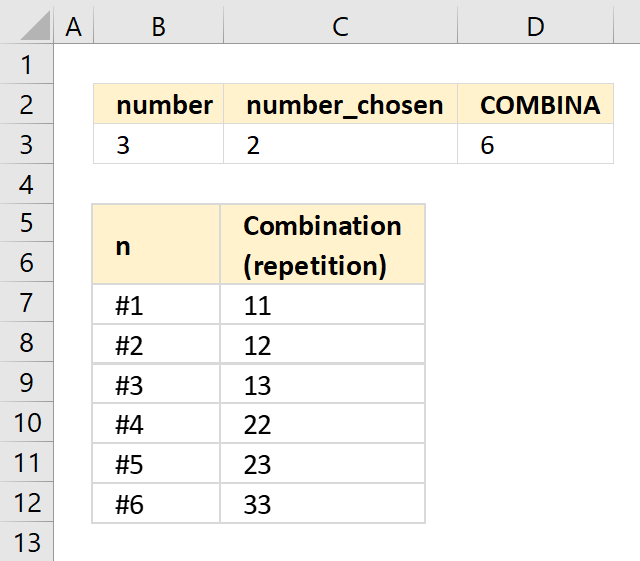Author: Oscar Cronquist Article last updated on October 01, 2018The COMBINA function calculates the number of combinations for a given number of elements from a larger group of elements.

Formula in cell D3:

=COMBINA(B3,C3)

Excel Function Syntax

COMBINA(number, number_chosen)

Arguments

 number Required. A number greater than or equal to zero and greater than or equal to Number_chosen. number_chosen Required. A number greater than or equal to 0.